Q.1) If 5^x-3^y=13438 and 5^(x-1)+3^(y+1)=9686 then find x+y.

a)13

b)14

[bg_collapse view=”button-blue” color=”#ffffff” expand_text=”Correct Answer” collapse_text=”Show Less” ]a)13[/bg_collapse] [bg_collapse view=”button-orange” color=”#4a4949″ expand_text=”View Solution” collapse_text=”Show Less” ]

Solution:

Here we can go by hit n trial

13438 is 5 digit number so 5^x must be a 5 digit number

5^6=15625

So putting x=6 in the first equation we get 3^y=2187

So y=7

Putting this in eq 2

5^5 +3^8=3125+6561=9686

So x+y=6+7=13

[/bg_collapse]

Q.2)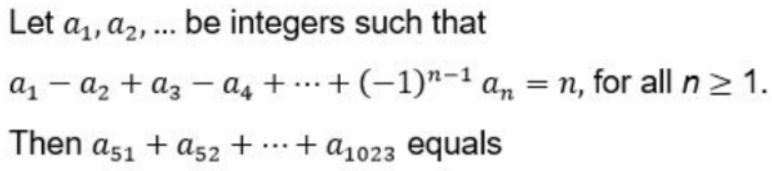a)0

b)10

c)1

d)-1

[bg_collapse view=”button-blue” color=”#ffffff” expand_text=”Correct Answer” collapse_text=”Show Less” ]c)1[/bg_collapse] [bg_collapse view=”button-orange” color=”#4a4949″ expand_text=”View Solution” collapse_text=”Show Less” ]

Solution:

a1=1

a1-a2=2

a2=-1

a1-a2+a3=3

a3=1

a1-a2+a3-a4=4

a4=-1

1,-1,1,-1….so on

so sum of every two consecutive terms will be 0

so only a1023 will remain which is odd numbered term  having value 1

[/bg_collapse]

Q.3) Amal invests Rs 12000 at 8% interest, compounded annually, and Rs 10000 at 6% interest, compounded semi-annually, both investments being for one year. Bimal invests his money at 7.5% simple interest for one year. If Amal and Bimal get the same amount of interest, then the amount, in Rupees, invested by Bimal is

a)20920

b)10920

[bg_collapse view=”button-blue” color=”#ffffff” expand_text=”Correct Answer” collapse_text=”Show Less” ]a)20920[/bg_collapse] [bg_collapse view=”button-orange” color=”#4a4949″ expand_text=”View Solution” collapse_text=”Show Less” ]

Solution:

Interests for both are same so equate their interests

Semi-annually for Amal so rate becomes half  (i.e.3% )and time will become double i.e. 2 years

Interest for Amal= (12000*8*1)/100 +10000[1.03]^2 -10000

Interest for Bimal= (P*7.5*1)/100

960+609=(7.5P)/100

156900=7.5P

P=20920

[/bg_collapse]

Q.4) Anil alone can do a job in 20 days while Sunil alone can do it in 40 days. Anil starts the job, and after 3 days, Sunil joins him. Again, after a few more days, Bimal joins them and they together finish the job. If Bimal has done 10% of the job, then in how many days was the job done?

a)14

b)15

c)13

d)12

[bg_collapse view=”button-blue” color=”#ffffff” expand_text=”Correct Answer” collapse_text=”Show Less” ]c)13[/bg_collapse] [bg_collapse view=”button-orange” color=”#4a4949″ expand_text=”View Solution” collapse_text=”Show Less” ]

Solution:

Work=lcm(20,40,3)=120

A=6 units per day

S=3 units per day

Anil does =6*3=18 units in 3 days

Bimal does 10% of the job=12 units

So anil and sunil will do 90% of the job=108 units

Anil  has already done 18 units

So anil and sunil will do 90 units in, Say X days

So

X(A+S)=90

Putting values of A and S

X=10 days

So B will also work for 10 days

So total=3+10=13 days were required to complete the work

[/bg_collapse]

Q.5)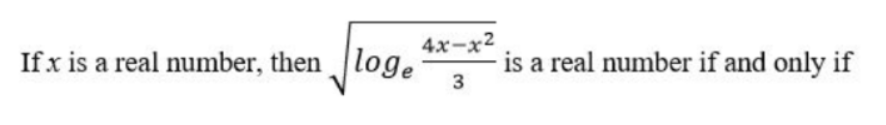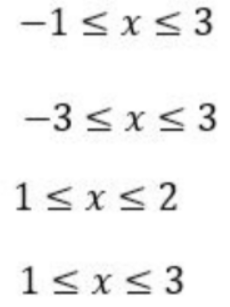a)1

b)2

c)3

d)4

[bg_collapse view=”button-blue” color=”#ffffff” expand_text=”Correct Answer” collapse_text=”Show Less” ]d)4[/bg_collapse] [bg_collapse view=”button-orange” color=”#4a4949″ expand_text=”View Solution” collapse_text=”Show Less” ]

Solution:

Using options, only option 4 satisfies because (4x-x^2)>0 for those values of x only

[/bg_collapse]

Q.6)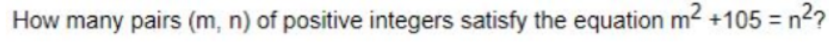a)4

b)5

[bg_collapse view=”button-blue” color=”#ffffff” expand_text=”Correct Answer” collapse_text=”Show Less” ]a)4[/bg_collapse] [bg_collapse view=”button-orange” color=”#4a4949″ expand_text=”View Solution” collapse_text=”Show Less” ]

Solution:

This can be rewritten as n^2-m^2=105

(n+m)(n-m)=105

We have an odd product in the RHS

So in LHS we must have only odd numbers that give a product of 105

Which are (1,105), (3,35), (5,21), (7,15)

So only 4 pairs will satisfy

iQuanta Shortcut: Number of factors/2= 8/2=4

[/bg_collapse]

Q.7) In 2010, a library contained a total of 11500 books in two categories – fiction and non-fiction. In 2015, the library contained a total of 12760 books in these two categories. During this period, there was 10% increase in the fiction category while there was 12% increase in the non-fiction category. How many fiction books were in the library in 2015?

a)6160

b)6600

c)6000

d)5500

[bg_collapse view=”button-blue” color=”#ffffff” expand_text=”Correct Answer” collapse_text=”Show Less” ]b)6600[/bg_collapse] [bg_collapse view=”button-orange” color=”#4a4949″ expand_text=”View Solution” collapse_text=”Show Less” ]

Solution:

Fiction….F

Non fiction….N

In 2010…..

F be the num of fiction books

11500-F be the number of non-fiction books

In 2015….

1.1F+1.12(11500-F)=12760

Calculating F in the equation above we get

F=6600

[/bg_colllapse]

Q.8) The salaries of Ramesh, Ganesh and Rajesh were in the ratio 6:5:7 in 2010, and in the ratio 3:4:3 in 2015. If Ramesh’s salary increased by 25% during 2010-2015, then the percentage increase in Rajesh’s salary during this period is closest to

a)9

b)8

c)7

d)10

[bg_collapse view=”button-blue” color=”#ffffff” expand_text=”Correct Answer” collapse_text=”Show Less” ]c)7[/bg_collapse] [bg_collapse view=”button-orange” color=”#4a4949″ expand_text=”View Solution” collapse_text=”Show Less” ]

Solution:

Salaries of Ramesh, Ganesh and Rajesh were  6x:5x:7x in 2010

Ratio of their salaries is 3y:4y:3y in 2015

Salary of Ramesh after 25% increase, salary=6x*1.25=7.5x

So 3y=7.5x

y=2.5x

so Rajesh’s salary in 2015=3y=7.5x

so % increase in Rajesh’s salary=(7.5x-7x)/7x *100=50/7=7.1% so 7% is closest.

[/bg_collapse]

Q.9)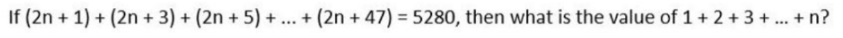a)4851

b)5851

[bg_collapse view=”button-blue” color=”#ffffff” expand_text=”Correct Answer” collapse_text=”Show Less” ]a)4851[/bg_collapse] [bg_collapse view=”button-orange” color=”#4a4949″ expand_text=”View Solution” collapse_text=”Show Less” ]

Solution:

From 1 to 47 we have 24 terms

48n+12(48)=5280

4n+48=440

4n=392

n=98

so 1+2+3+4+…..+98

98/2(99)=49*99=4851

[/bg_collapse]

Q.10) Two circles, each of radius 4 cm, touch externally. Each of these two circles is touched externally by a third circle. If these three circles have a common tangent, then the radius of the third circle, in cm, is

a)1

b)2

c)4

d)5

[bg_collapse view=”button-blue” color=”#ffffff” expand_text=”Correct Answer” collapse_text=”Show Less” ]a)1[/bg_collapse] [bg_collapse view=”button-orange” color=”#4a4949″ expand_text=”View Solution” collapse_text=”Show Less” ]

Solution:

r is the radius of the smaller circle.

In right triangle ABC

AB^2+BC^2=AC^2

(4-r)^2+4^2=(4+r)^2

r=1

[/bg_collapse]

Q.11) Let A and B be two regular polygons having a and b sides, respectively. If b = 2a and each the interior angle of B is 3/2 times each interior angle of A, then each interior angle, in degrees, of a regular polygon with a + b sides is

a)140

b)150

[bg_collapse view=”button-blue” color=”#ffffff” expand_text=”Correct Answer” collapse_text=”Show Less” ]b)150[/bg_collapse] [bg_collapse view=”button-orange” color=”#4a4949″ expand_text=”View Solution” collapse_text=”Show Less” ]

Solution:

the angle of B=1.5 * angle of A

so the angle of B is greater

(b-2)*180/b=1.5*(a-2)*180/a

(b-2)/b=1.5(a-2)/a

ab-2a=1.5ab-3b

0.5ab=3b-2a

½=3/a-2/b

b=2a

so a=4….b=8

a+b=12

so each interior angle of polygon having 12 sides is (12-2)*180/12=150

[/bg_collapse]

Q.12) John jogs on track A at 6 kmph and Mary jogs on track B at 7.5 kmph. The total length of tracks A and B is 325 metres. While John makes 9 rounds of track A, Mary makes 5 rounds of track B. In how many seconds will Mary make one round of track A?

a)34

b)48

c)45

d)50

[bg_collapse view=”button-blue” color=”#ffffff” expand_text=”Correct Answer” collapse_text=”Show Less” ]b)48[/bg_collapse] [bg_collapse view=”button-orange” color=”#4a4949″ expand_text=”View Solution” collapse_text=”Show Less” ]

Solution:

A+B=325…eq1

9A/6=5B/7.5

A=4B/9

So putting in eq1

4B/9+B=325

B=225m

A=100 m

Speed of Mary=7.5 kmph=37.5/18  m/sec

So Mary completes one round of A i.e 100 m in =100/(37.5/18)=18000/375=48 sec

[/bg_collapse]

Q.13) In a triangle ABC, medians AD and BE are perpendicular to each other, and have lengths 12 cm and 9 cm, respectively. Then, the area of triangle ABC, in sq cm, is

a)50

b)60

c)72

d)80

[bg_collapse view=”button-blue” color=”#ffffff” expand_text=”Correct Answer” collapse_text=”Show Less” ]c)72[/bg_collapse] [bg_collapse view=”button-orange” color=”#4a4949″ expand_text=”View Solution” collapse_text=”Show Less” ]

Solution: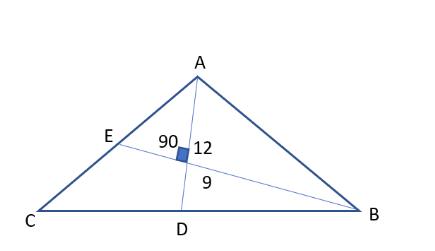Since median intersect at centroid and centroid divides median into 2:1

So

AG=8….GD=4

BG=6…GE=3

So Area of ABC=2*36=72

[/bg_collapse]

Q.14)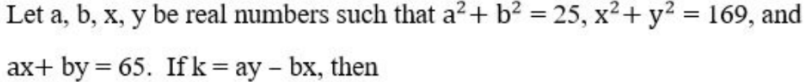a)k>5/7

b)k<=5/7

c)k=0

d)None of these

[bg_collapse view=”button-blue” color=”#ffffff” expand_text=”Correct Answer” collapse_text=”Show Less” ]c)k=30[/bg_collapse] [bg_collapse view=”button-orange” color=”#4a4949″ expand_text=”View Solution” collapse_text=”Show Less” ]

Solution:

a,b=+-3, +-4

x,y=+-12, +-5

But no combination of these values gives us 65

But a=5, b=0…x=13….y=0

This satisfies ax+by=65

So k=0

[/bg_collapse]

Q.15)The base of a regular pyramid is a square and each of the other four sides is an equilateral triangle, length of each side being 20 cm. The vertical height of the pyramid, in cm, is

a)10√2

b)10

c)20

d)20√2

[bg_collapse view=”button-blue” color=”#ffffff” expand_text=”Correct Answer” collapse_text=”Show Less” ]a)10√2[/bg_collapse] [bg_collapse view=”button-orange” color=”#4a4949″ expand_text=”View Solution” collapse_text=”Show Less” ]

Solution: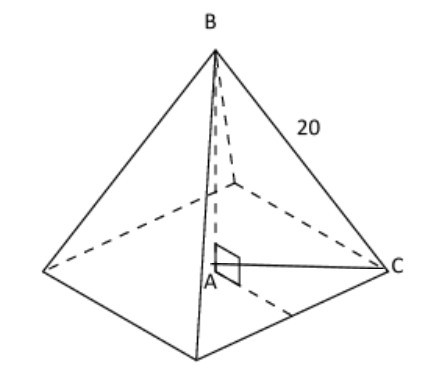Since each side is an equilateral triangle of side 20 cm ,

Hence the side of base square will also be 20 each.

So in right triangle ABC

BC^2=AB^2+AC^2

AC= ½ * diagonal of square

Diagonal of square= 20√2

So AC=10√2

So AB^2=20^2-(10√2)^2=200

AB=10√2

[/bg_collapse]

Q.16) The quadratic equation x^2+ bx + c = 0 has two roots 4a and 3a, where a is an integer. Which of the following is a possible value of b^2+ c?

a)427

b)549

c)366

d)305

[bg_collapse view=”button-blue” color=”#ffffff” expand_text=”Correct Answer” collapse_text=”Show Less” ]b)549[/bg_collapse] [bg_collapse view=”button-orange” color=”#4a4949″ expand_text=”View Solution” collapse_text=”Show Less” ]

Solution:

So roots will be 4a,3a

So equation will be x^2-7ax+12a^2=0

b^2+c=(7a)^2+12a=49a^2+12a^2=61a^2

so answer must be a multiple of 61 multiplied by a perfect square which is only among the 549

[/bg_collapse]

Q.17) Two ants A and B start from a point P on a circle at the same time, with A moving clock-wise and B moving anti-clockwise. They meet for the first time at 10:00 am when A has covered 60% of the track. If A returns to P at 10:12 am, then B returns to P at

a)10:14

b)10:27

c)10:15

d)10:29

[bg_collapse view=”button-blue” color=”#ffffff” expand_text=”Correct Answer” collapse_text=”Show Less” ]b)10:27[/bg_collapse] [bg_collapse view=”button-orange” color=”#4a4949″ expand_text=”View Solution” collapse_text=”Show Less” ]

Solution: